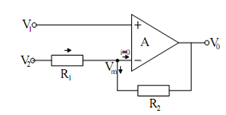## Basic Analog Circuits using Ideal Op-amps Assignment Help

Assignment Help: >> Operational Amplifiers and their Applications - Basic Analog Circuits using Ideal Op-amps

Basic Analog Circuits using Ideal Op-amps:

In the following, we illustrates different controlled sources and other useful circuits, which may be realized by using IC op-amps.

VCVS Circuits

Assume the 3-port VCVS of Figure. Supposing ideal op-amp (i.e. Zin = ∞, Z0 = 0, A → ∞) the node equation at node m is

V2  - Vm /R1 = Vm  - V0 /R2Figure: The 3-port VCVS

Solving for Vm gives,

Vm   =  (R2/ R1 + R2 )V  + (R1/ R1 + R2)V0

Also,

(V1 - Vm ) A = V0

or (V1  - V m ) = V 0 /A

which means V1 = Vm as A → ∞

From (50) and (52), we determine

V0  =  (1 +( R2 / R1) )V1  - (R2 / R1)V2

 Current Controlled Current Sources Current Controlled Voltage Sources Effect of Op-amp Pole Finite Gain Differential Amplifiers Inverting Amplifier Inverting Differentiator Inverting Integrator Negative Impedance Converter Voltage-Controlled Current Sources